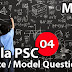## Recent Posts

1. Which of the following numbers of divisible by 33
[a] 1333
[b] 1375
[c] 2133
[d] 2145

To divide a number with 33, it must be
completely divide with its factors 11
and 3
2145 is completely divisible by 3 because
sum of the digits 2+1+4+5 12, which is a
multiple of 3
2145 is also divisible by 11
2+4-1+5 = 6-6=0

2. The sum and product of two numbers are 13 and 40 respectively. The sum of their reciprocals is:
[a] 1/40
[b] 4/40
[c] 13/40
[d] 30/40

 sum of Reciprocals = sum of numbers product of numbers
13
40

3. Difference between two numbers is 6 and difference between their squares is 180. Find the sum of two numbers.
[a] 30
[b] 32
[c] 34
[d] 36

x² - y² = (x+y) (x-y)
180 = (x+y)x6
x+y = 180/6 = 30

4. The sum of two numbers is 18 and their product is 72. The smallest number
[a] 5
[b] 6
[c] 7
[d] 8

6+12 → 18, 6x12 → 72
smallest number → 6

5. Find the least positive number which when added to 962 will make it a perfect square
[a] 64
[b] 127
[c] 62
[d] 95

Next perfect square is 1024
i.e. 962 + 62

6. There are 3 numbers. The product of the first and second is 700. The product of second and third is 480. The product of first and third is 840. Find the numbers.
[a] 25, 20, 34
[b] 42, 20, 24
[c] 70, 10, 48
[d] 35, 20, 24

35 x 20 = 700,
20 x 24 = 480,
35 x 24 = 840

7. The denary number equal to (1011)2
[a] 10
[b] 11
[c] 8
[d] 12

1x23 + 0x22 + 1x21 + 1x20
8+0+2+1 = 11

8. What are the last two digits of the number 745.
[a] 07
[b] 23
[c] 49
[d] 43

71 = 7, 72 = 49,
73 = 343, 74 = 2401
75 = 16807, 76 =117649

9. What will be the least number which when doubled will be exactly divisible 24, 18, 21 and 10
[a] 2520
[b] 1260
[c] 630
[d] 5040

LCM of 24, 18, 21, 10 → 2520 smallest number which can divided
by 24, 18, 21, 10 is
LCM/2
i.e. 2520/2 = 1260

10. The smallest perfect square which is exactly divisible by 2, 3, 4, 5 and 6.
[a] 1600
[b] 900
[c] 784
[d] 1800

LCM of 2, 3, 4, 5, 6 → 60 smallest perfect square in the
option which can be divided by
60 is 900

Kerala PSC GK | Practice/Model Maths Questions - 4Reviewed by Santhosh Nair on 12:05 PM Rating: 5